• 以下算法的时间复杂度为
千次阅读
2019-03-19 21:02:21

### 一、算法复杂度

算法是为求解一个问题需要遵循的、被清楚指定的简单指令的集合，简单来说就是解决特定问题求解步骤的描述。对于一个问题，一旦某种算法给定并且是正确的，那么重要的一步，就是确定该算法将需要多少时间或者空间等资源量的问题。如果一个问题的求解算法竟然需要长达一年的时间，那么这种算法就很难有什么用处了，同样，一个需要若干个GB内存的算法在当前大多数机器上也是无法使用的。

算法复杂度分为时间复杂度和空间复杂度

• 时间复杂度是指执行这个算法所需要的计算工作量
• 空间复杂度是指执行这个算法所需要的内存空间

### 二、时间复杂度

1.时间复杂度的概念

首先分析算法的时间复杂度，使用大O表示法，其核心思想是：所有代码的执行时间与代码的执行次数成正比，可以使用以下公式来表达：

                                T(n) = O( f(n) )

对该公式的解释如下：

• T(n) 表示算法中语句的执行次数
• n 表示数据规模的大小，数据规模n不同，代码的执行时间T(n)也会随之而改变
• f(n)  算法的基本操作(执行次数最多的那条语句)重复执行的次数

在进行算法分析时，代码的总执行时间T(n)是关于问题规模n的函数，进而分析T(n)随n的变化情况并确定T(n)的数量级。算法的时间复杂度，记作：T(n)= O(f(n))。它表示随问题规模n的增大，算法执行时间的增长率和f(n)的增长率相同，大O时间复杂度实际上并不具体表示代码的真正执行时间，而是表示代码执行时间随数据规模增长的变化趋势，所以称作算法的渐近时间复杂度，简称为时间复杂度。

2.分析时间复杂度的过程

2.1 计算时间复杂度遵守的守则：

（1）对于一些简单的输入输出语句或赋值语句,近似认为需要O(1)时间，（注意：常数项忽略不计）

（2）对于顺序结构,需要依次执行一系列语句所用的时间可采用大O下"求和法则"

加法原则:总复杂度等于量级最大的代码复杂度，即忽略低阶项，高阶项系数以及常数项

因此，我们只关注循环次数最多的代码

（3）对于选择结构,如if语句,它的主要时间耗费是在执行then字句或else字句所用的时间,需注意的是检验条件也需要O(1)时间

（4）对于循环结构,循环语句的运行时间主要体现在多次迭代中执行循环体以及检验循环条件的时间耗费,一般可用大O下"乘法法则"

乘法原则：嵌套代码的复杂度等于嵌套内外代码的复杂度乘积

（5）对于复杂的算法,可以将它分成几个容易估算的部分,然后利用求和法则和乘法法则技术整个算法的时间复杂度

2.2 计算时间复杂度步骤：

第一步：找出算法中的基本语句（执行次数最多的那条语句），通常是最内层循环的循环体。

第二步：计算基本语句的执行次数的数量级

第三步：将基本语句执行次数的数量级放入大Ο记号中

2.3常见时间复杂度举例

2.3.1 常数阶0(1)

int a=3;
int b=4;
int sum=a+b;

总结：只要代码的执行时间不随n的增大而增大，这样的代码时间复杂度都为O(1），一般情况下，只要代码中不存在循环语句、递归语句，即使代码成千上万，都是常数阶

2.3.2 线性阶O(n)

 int sum=0；
for(i=0;i<n;i++){
sum++;
}

2.3.3平方阶O(n^2）

最常见的平方阶算法即为两个循环嵌套的情况

int i;
for(i = 0 ; i < n ; i++){
for(j = 0 ; j < n ; j++){
System.out.println("hello");
}
}

以下这段代码的时间复杂度同样为平方阶

int i;
for(i = 0 ; i < n ; i++){
for(j = i ; j < n ; j++){
System.out.println("hello");
}
}

这段代码的内循环 j 是从i开始的，而不是从0开始，因此它的总执行时间为：n+(n-1)+(n-2)+...+1 =n^2/2+n/2

忽略低阶项，去掉最高阶系数，得出它的时间复杂度为0(n^2)

2.3.4 对数阶O(logn)

int i = 1;
while(i <= n){
i *= 2;
}

这段代码表示：当 x个2相乘大于n时，退出循环，即2^x=n    x=log2(n)  ,代码执行次数x为log2(n),

2.3.5 线性对数阶 O(nlogn)


for(int i = 0 ; i < n ; i++){
fun (i);
}

void fun(int n){
while(i<n){
i*=2;
}
}

外层循环调用的fun()函数时间复杂度为O(logn），利用乘法原则，代码的时间复杂度为O(nlogn)

2.4常见时间复杂度比较

O(1) < O(logn)  < O(n)  < O(nlogn) < O(n^2) < O(n^3) < { O(2^n) < O(n!) < O(n^n) }

注意： O(2^n)     O(n!)     O(n^n) 这三项都是非多项式时间复杂度

当N越来越大时，它们的时间复杂度会急剧增长，因此，算法时间复杂度为以上三者时，算法效率奇低

### 三、空间复杂度

空间复杂度：一个算法在运行过程中临时占用存储空间大小的量度，记做S(n)=O(f(n))，其中，n为问题的规模，f(n)为语句关于n所占存储空间的函数。

常见空间复杂度： O(1)   O(n)

对于一个算法，其时间复杂度和空间复杂度往往是相互影响的。当追求一个较好的时间复杂度时，可能会使空间复杂度的性能变差，即可能导致占用较多的存储空间；反之，当追求一个较好的空间复杂度时，可能会使时间复杂度的性能变差，即可能导致占用较长的运行时间。

更多相关内容
• 算法时间复杂度和空间复杂度合称为算法的复杂度。 ​ 时间复杂度时间复杂度是指执行算法所需要的计算工作量； ​ 空间复杂度： 是对一个算法在运行过程中临时占用存储空间大小的量度； 算法的复杂性体运行该...

# 1.算法的复杂度：

算法的时间复杂度和空间复杂度合称为算法的复杂度。

时间复杂度： 时间复杂度是指执行算法所需要的计算工作量；

空间复杂度： 是对一个算法在运行过程中临时占用存储空间大小的量度；

算法的复杂性体运行该算法时的计算机所需资源的多少上，计算机资源最重要的是时间和空间（即寄存器）资源，因此复杂度分为时间和空间复杂度。

# 2.时间复杂度：

一个算法花费的时间与算法中语句的执行次数成正比例，哪个算法中语句执行次数多，它花费时间就多。一个算法中的语句执行次数称为语句频度或时间频度，记为T(n)。

一般情况下，算法中基本操作重复执行的次数是问题规模n的某个函数，用T(n)表示，若有某个辅助函数f(n)，使得当n趋近于无穷大时，T(n)/f (n)的极限值为不等于零的常数，则称f(n)是T(n)的同数量级函数。记T(n)=O(f(n))，称O(f(n)) 为算法的渐进时间复杂度，简称时间复杂度。## 1.大O表示法：

用O( )来体现算法时间复杂度的记法，我们称之为大O表示法。

算法复杂度可以从最理想情况、平均情况和最坏情况三个角度来评估，由于平均情况大多和最坏情况持平，而且评估最坏情况也可以避免后顾之忧，因此一般情况下，我们设计算法时都要直接估算最坏情况的复杂度。大O表示法所表示的是一个算法在最糟糕情况下的运行时间。

## 2.大O推导方法：

1.用常数1来取代运行时间中所有加法常数。

2.修改后的运行次数函数中，只保留最高阶项

3.最高项除去其相乘的常数，得到的结果就是大O阶。

举例：

单个循环体情况：

void Demo(int n){
for(int j = 0; j < n; j++) {        // 循环次数为 n
printf("Hello, World!\n");      // 循环体时间复杂度为 O(1)
}
}
//时间复杂度为 O(n × 1)，即 O(n)。


多重循环体情况：

void Demo(int n) {
for(int i = 0; i < n; i++) {            // 循环次数为 n
for(int j = 0; j < n; j++) {        // 循环次数为 n
printf("Hello, World!\n");      // 循环体时间复杂度为 O(1)
}
}
}
//时间复杂度为 O(n × n × 1)，即 O(n^2)。


多个事件复杂度情况：

void Demo(int n) {
for(int i = 0; i < n; i++) {
for(int j = 0; j < n; j++) {
printf("Hello, World!\n");// 第一部分时间复杂度为 O(n^2)
}
}
for(int j = 0; j < n; j++) {
printf("Hello, World!\n");// 第二部分时间复杂度为 O(n)
}
}
//时间复杂度为 max(O(n^2)，O(n))，即 O(n^2)。


## 3.一些常见的大O运行时间：

• O(log n)，对数时间，二分查找。
• O(n)，线性时间，简单查找。
• O(n logn)，快速排序——速度较快的排序算法。
• O(n²)，选择排序——速度较慢的排序算法。
• O(n!)，旅行商问题的解决方案——非常慢的算法。

# 3.空间复杂度：

一个程序的空间复杂度是指运行完一个程序所需内存的大小，利用程序的空间复杂度，可以对程序的运行所需要的内存多少有个预先估计。一个程序执行时除了需要存储空间和存储本身所使用的指令、常数、变量和输入数据外，还需要一些对数据进行操作的工作单元和存储一些为现实计算所需信息的辅助空间。程序执行时所需存储空间包括以下两部分。

（1）固定部分：这部分空间的大小与输入/输出的数据的个数多少、数值无关，主要包括指令空间（即代码空间）、数据空间（常量、简单变量）等所占的空间，这部分属于静态空间。

（2）可变空间：这部分空间的主要包括动态分配的空间，以及递归栈所需的空间等，这部分的空间大小与算法有关。一个算法所需的存储空间用f(n)表示。S(n)=O(f(n))，其中n为问题的规模，S(n)表示空间复杂度。

通常来说，只要算法不涉及到动态分配的空间以及递归、栈所需的空间，空间复杂度通常为0(1)。

# 4.个别特殊举例：

## 1.斐波那契数列的时间和空间复杂度

//递归情况下的斐波那契数列
public static int Fib(int num){
if (num==1||num==2){
return num;
}
return Fib(num-2)+Fib(num-1);
}


递归的时间复杂度是： 递归次数*每次递归中执行基本操作的次数，所以，时间复杂度是： O(2^N)。

递归的空间复杂度是： 递归的深度*每次递归所需的辅助空间的个数，所以，空间复杂度是：O(N)。

## 2.二分法的时间复杂度和空间复杂度

二分查找在最坏的情况下依次是n/2,n/4,n/8一直到1为止。
假设是x次，然后我们可以观察到分母是每次都乘以1/2，分子不变，所以可以列出下面等式：

n(1/2)^x = 1

通过计算得出x=log2N，即时间复杂度为O(log2N);

非递归：

public static int binarySearch(int[] arr,int toFind) {
int left = 0;
int right = arr.length-1;
while (left <= right) {
int mid = (left + right) / 2;
if (arr[mid] < toFind) {
left = mid + 1;
}
else if (arr[mid] > toFind) {
right = mid - 1;
}
else {
return mid;
}
}
return -1;
}


时间复杂度：循环的基本次数是log2N，所以，时间复杂度是O(log2N);

空间复杂度：由于辅助空间是常数级别的，所以，空间复杂度是O(1)。

递归：

public static int binarySearch(int srcArray[], int start, int end, int key) {
int mid = (end - start) / 2 + start;
if (srcArray[mid] == key) {
return mid;
}
if (start >= end) {
return -1;
} else if (key > srcArray[mid]) {
return binarySearch(srcArray, mid + 1, end, key);
} else if (key < srcArray[mid]) {
return binarySearch(srcArray, start, mid - 1, key);
}
return -1;
}


递归的次数和深度都是log2N,每次所需要的辅助空间都是常数级别的：
时间复杂度 : O(log2N)
空间复杂度：O(log2N)

展开全文java 开发语言 算法
• 算法复杂度——时间复杂度时间复杂度概念定义 时间复杂度 概念定义

# 时间复杂度

## 概念定义

根据定义，时间复杂度指输入数据大小为 N 时，算法运行所需花费的时间。需要注意：

1. 统计的是算法的「计算操作数量」，而不是「运行的绝对时间」。计算操作数量和运行绝对时间呈正相关关系，并不相等。算法运行时间受到「编程语言 、计算机处理器速度、运行环境」等多种因素影响。例如，同样的算法使用 Python 或 C++ 实现、使用 CPU 或 GPU 、使用本地 IDE 或力扣平台提交，运行时间都不同。
2. 体现的是计算操作随数据大小 N 变化时的变化情况。假设算法运行总共需要「 1 次操作」、「 100 次操作」，此两情况的时间复杂度都为常数级 O(1) ；需要「 N 次操作」、「 100N 次操作」的时间复杂度都为 O(N)。

## 符号表示

根据输入数据的特点，时间复杂度具有「最差」、「平均」、「最佳」三种情况，分别使用 O, Θ, Ω 三种符号表示。以下借助一个查找算法的示例题目帮助理解。
题目： 输入长度为 N 的整数数组 nums ，判断此数组中是否有数字7，若有则返回 true ，否则返回 false。
解题算法： 线性查找，即遍历整个数组，遇到 7 则返回 true 。
Python代码：

def find_seven(nums):
for num in nums:
if num == 7:
return True
return False

• 最佳情况 Ω(1) ： nums = [7, a, b, c, …] ，即当数组首个数字为 7 时，无论 nums 有多少元素，线性查找的循环次数都为 1 次；
• 最差情况 O(N) ： nums = [a, b, c, …] 且 nums 中所有数字都不为 7 ，此时线性查找会遍历整个数组，循环 N 次；
• 平均情况 Θ ： 需要考虑输入数据的分布情况，计算所有数据情况下的平均时间复杂度；例如本题目，需要考虑数组长度、数组元素的取值范围等；

O 是最常使用的时间复杂度评价渐进符号，下文示例与本 LeetBook 题目解析皆使用 O

## 常见种类

根据从小到大排列，常见的算法时间复杂度主要有：

O(1) < O(log N) < O(N) < O(N log N) < O(N 2) < O(2N) < O(N !)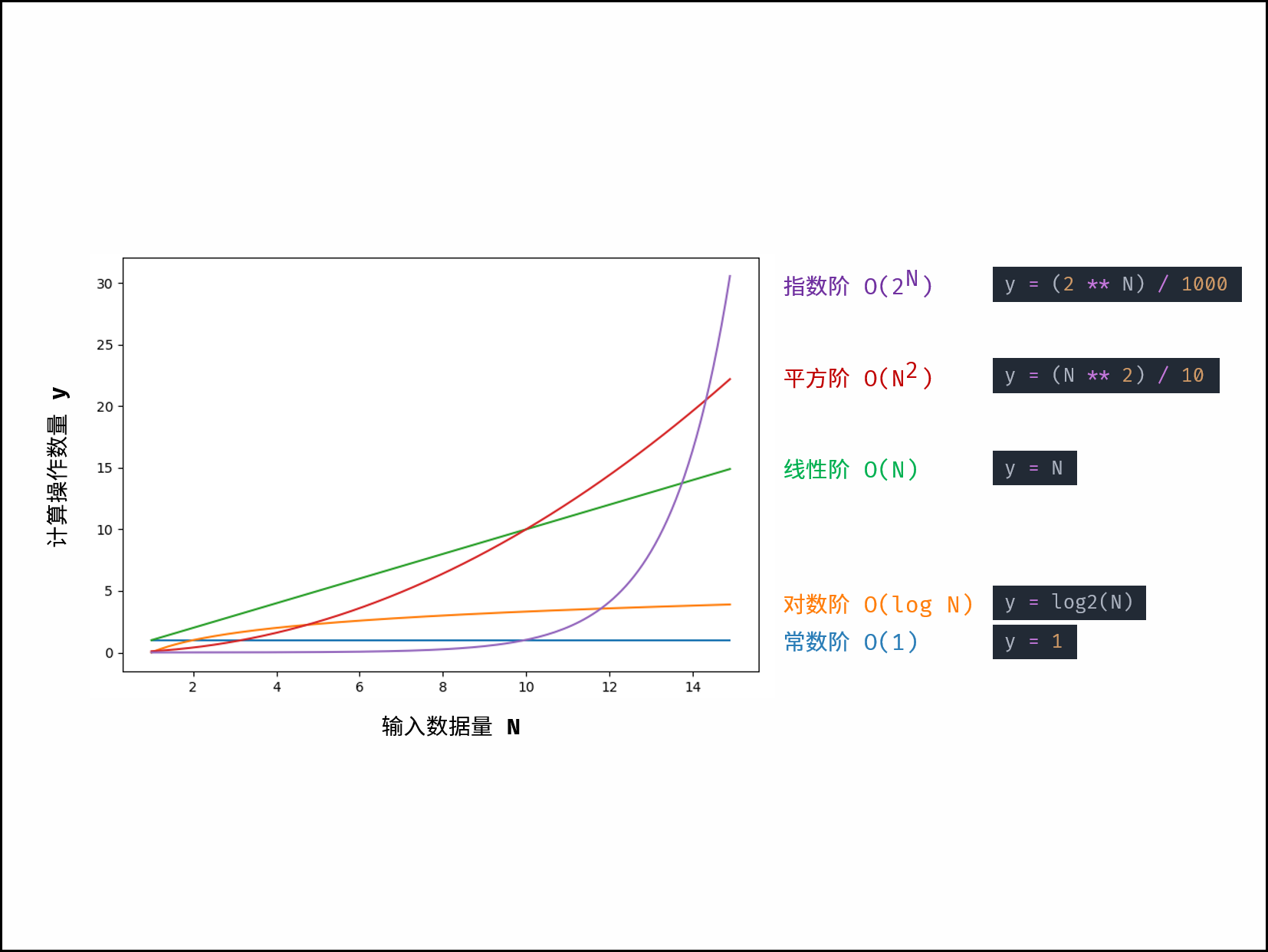## 示例解析

对于以下所有示例，设输入数据大小为 N ，计算操作数量为 count 。图中每个「蓝色方块」代表一个单元计算操作。

### 常数O(1) ：

运行次数与 N 大小呈常数关系，即不随输入数据大小 N 的变化而变化。

def algorithm(N):
a = 1
b = 2
x = a * b + N
return 1


对于以下代码，无论 a 取多大，都与输入数据大小 N 无关，因此时间复杂度仍为 O(1) 。

def algorithm(N):
count = 0
a = 10000
for i in range(a):
count += 1
return count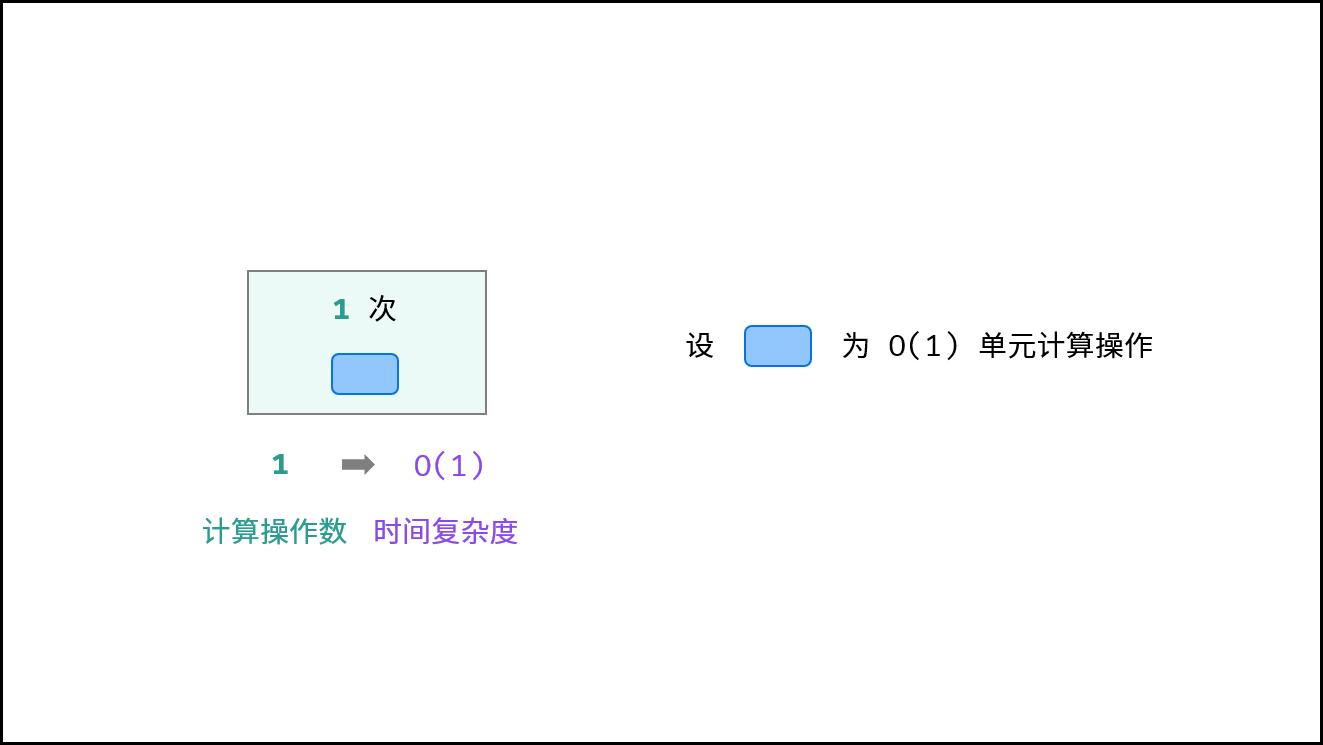### 线性O(N)：

循环运行次数与N大小呈线性关系，时间复杂度为O(N)。

def algorithm(N):
count = 0
for i in range(N):
count += 1
return count


对于以下代码，虽然是两层循环，但第二层与N大小无关，因此整体仍与N呈线性关系。

def algorithm(N):
count = 0
a = 10000
for i in range(N):
for j in range(a):
count += 1
return count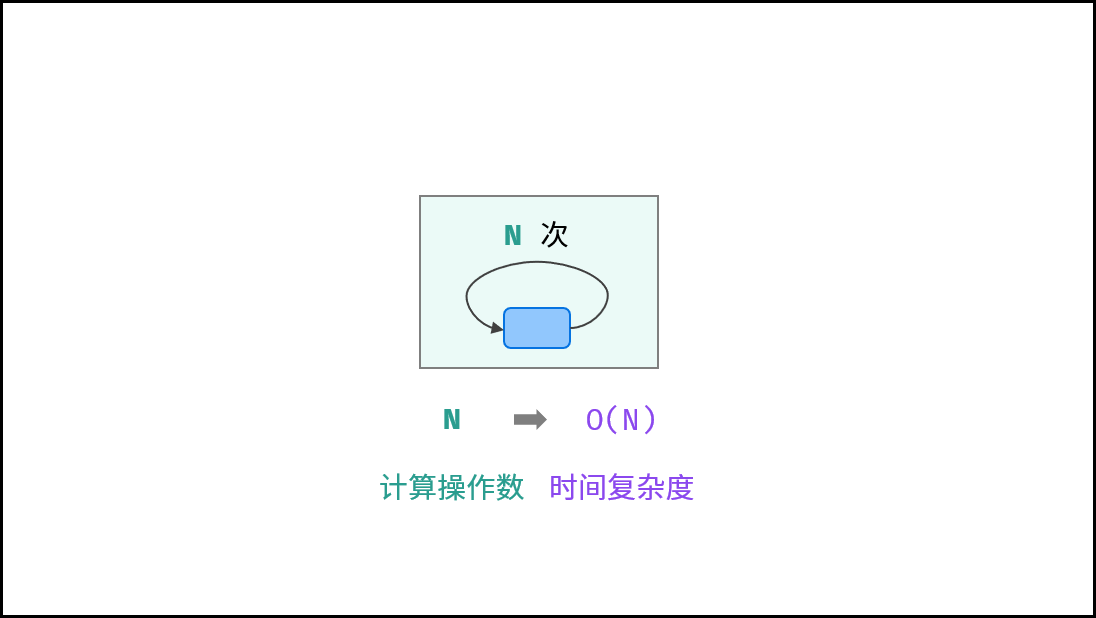### 平方O(N2)：

两层循环相互独立，都与N呈线性关系，因此总体与N呈平方关系，时间复杂度为O(N 2)。

def algorithm(N):
count = 0
for i in range(N):
for j in range(N):
count += 1
return count


冒泡排序为例，其包含两层独立循环：

1. 第一层复杂度为O (N)；
2. 第二层平均循环次数为N/2，复杂度为O(N )，推导过程如下：
O(N/2) = O(1/2)O(N) = O(1)O(N) = O(N)
因此，冒泡排序的总体时间复杂度为O(N 2)，代码如下所示：
def bubble_sort(nums):
N = len(nums)
for i in range(N - 1):
for j in range(N - 1 - i):
if nums[j] > nums[j + 1];
nums[j], nums[j + 1] = nums[j + 1], nums[j]
return nums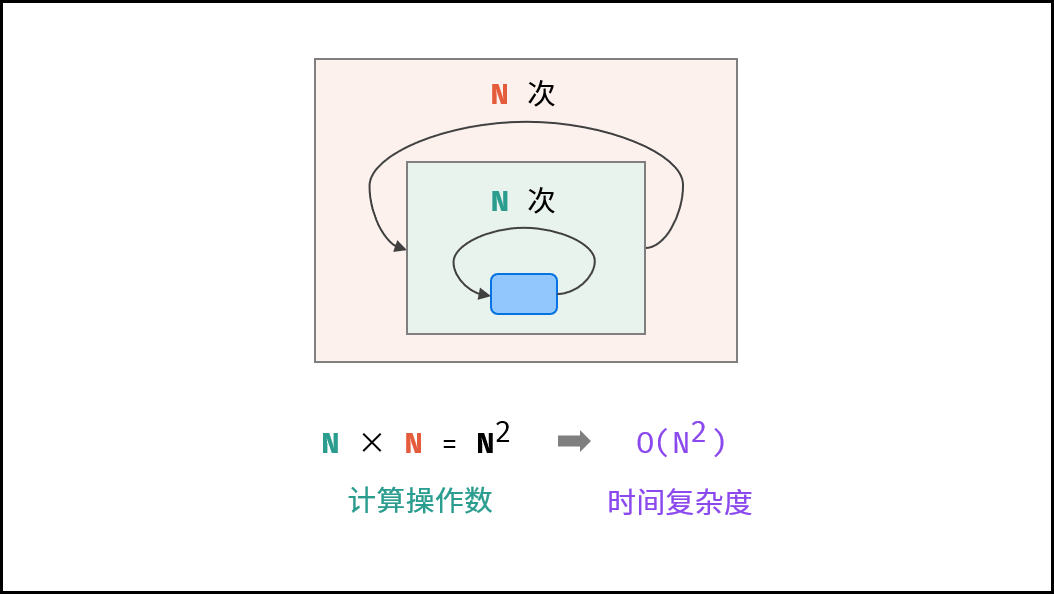### 指数O(2N)：

生物学科中的“细胞分裂”即是指数级增长。初始状态为1个细胞，分裂一轮后为2个，分裂两轮后为4个，……，分裂N轮后有2N个细胞。
算法中，指数阶常出现与递归，算法原理图与代码如下所示：

def algorithm(N)
if N <= 0: return 1
count_1 = algorithm(N - 1)
count_2 = algorithm(N - 1)
return count_1 + count_2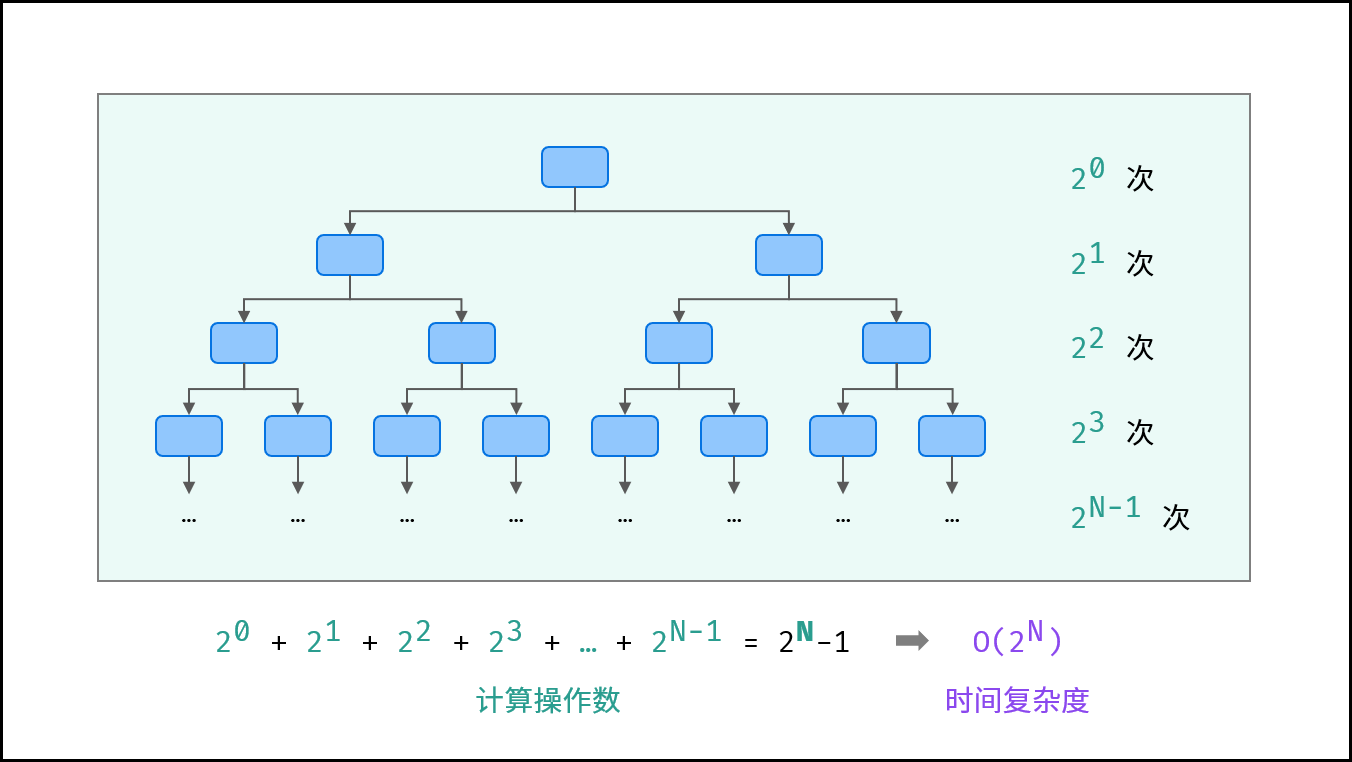### 阶乘O(N !)：

阶乘阶对应数学上常见的“全排列”。即给定N个互不重复的元素，求其所有可能的排列方案，则方案数量为：
N×(N−1)×(N−2)×⋯×2×1=N !
如下图与代码所示，阶乘常使用递归实现，算法原理：第一层分裂出N个，第二层分裂出N-1个，……，直至到第N层时终止并回溯。

def algorithm(N):
if N <= 0: return 1
count = 0
for _ in range(N):
count += algorithm(N - 1)
return count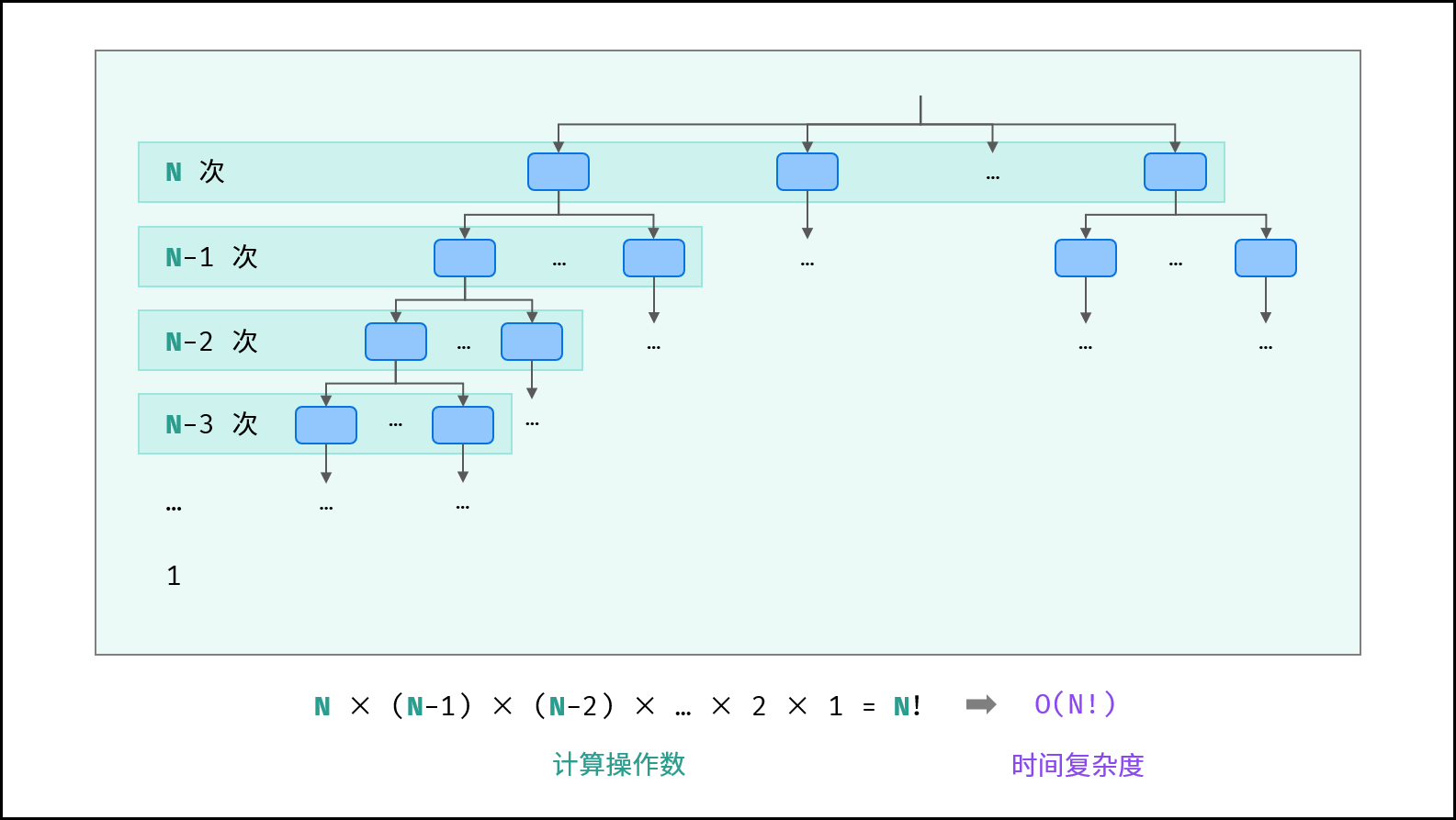### 对数O(logN)：

对数阶与指数阶相反，指数阶为“每轮分裂出两倍的情况”，而对数阶是“每轮排除一半的情况”。对数阶常出现于二分法分治等算法中， 体现着“一分为二”或“一分为多”的算法思想。
设循环次数为m，则输入数据大小N与2m呈线性关系，两边同时取log2对数，则得到循环次数m与log2N呈线性关系，即时间复杂度为O(logN)。

def algorithm(N):
count = 0
i = N
while i > 1:
i = i / 2
count += 1
return count


如以下代码所示，对于不同 a 的取值，循环次数 m 与 loga N 呈线性关系 ，时间复杂度为 O(loga N)。而无论底数 a 取值，时间复杂度都可记作 O(logN) ，根据对数换底公式的推导如下：

O(loga N) = O(log2 N)/O(log2 a) = O(log N)

def algorithm(N):
count = 0
i = N
a = 3
while i > 1:
i = i / a
count += 1
return count


如下图所示，为二分查找的时间复杂度示意图，每次二分将搜索区间缩小一半。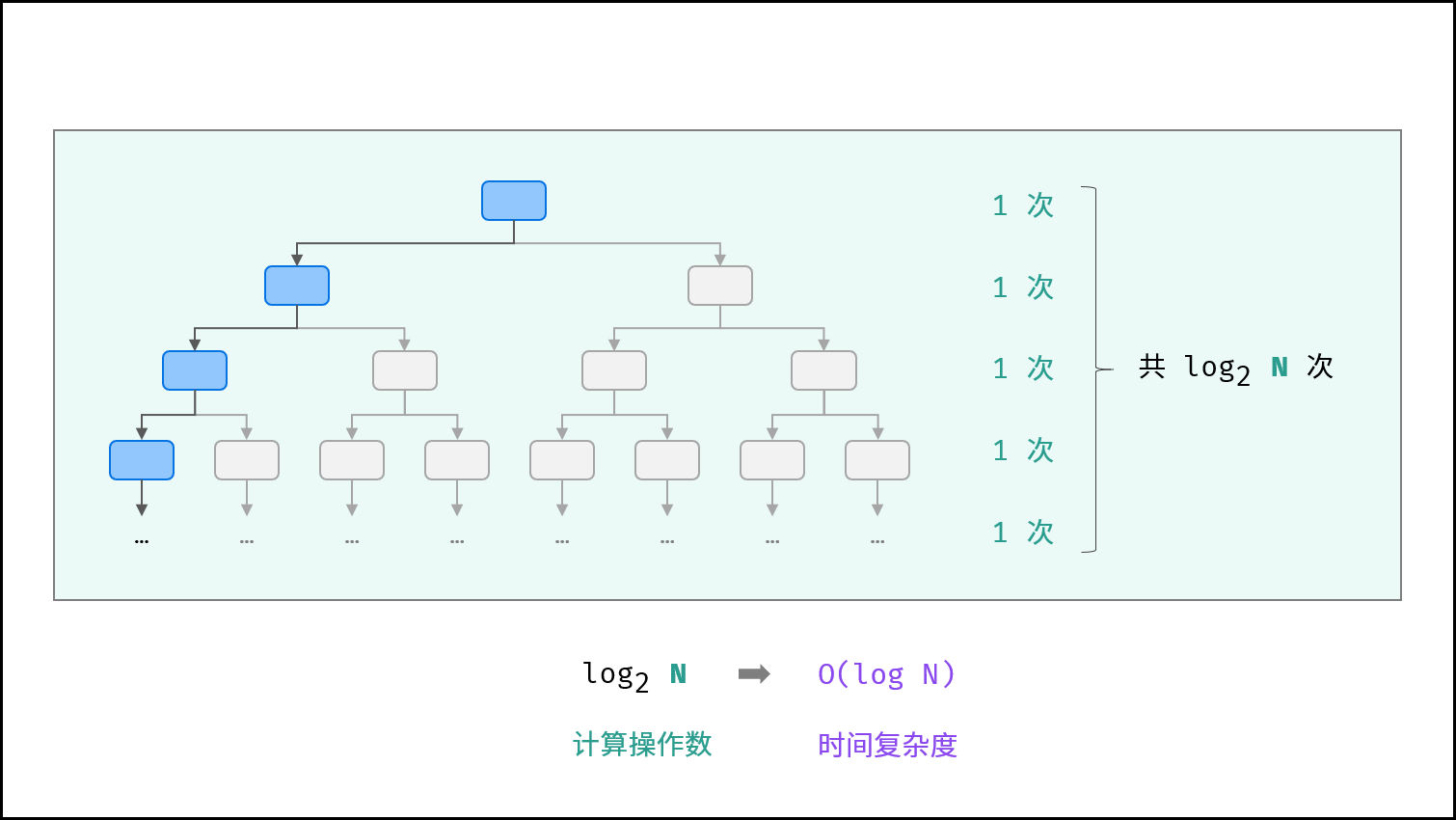### 线性对数O(N log N)：

两层循环相互独立，第一层和第二层时间复杂度分别为 O(log N) 和 O(N) ，则总体时间复杂度为 O(N logN) ；

def algorithm(N):
count = 0
i = N
while i > 1:
i = i / 2
for j in range(N):
count += 1
return count


线性对数阶常出现于排序算法，例如「快速排序」、「归并排序」、「堆排序」等，其时间复杂度原理如下图所示。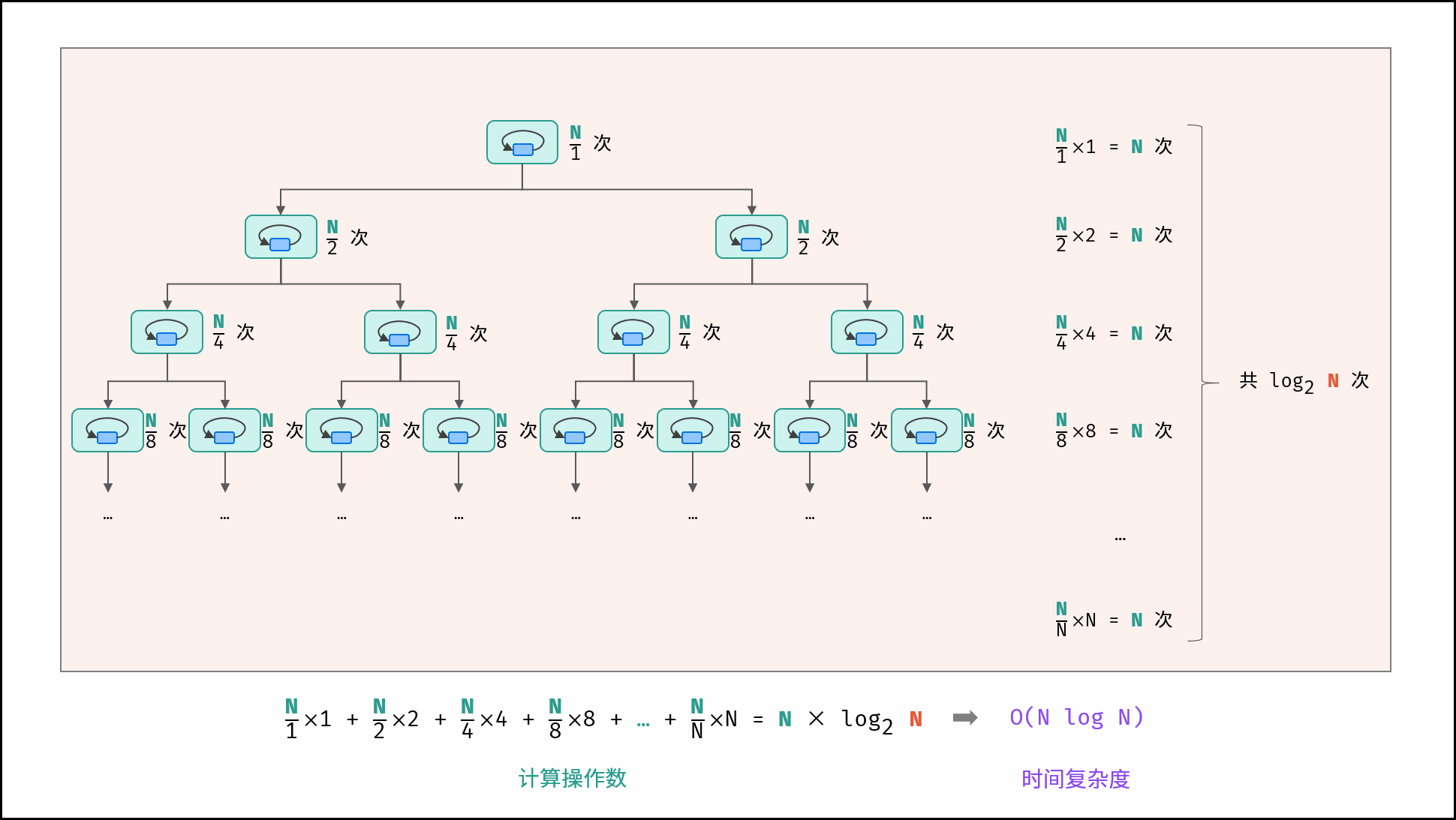展开全文算法 排序算法 leetcode python
• 算法复杂度分为时间复杂度和空间复杂度。 时间复杂度用于度量算法执行的时间长短；而空间复杂度则是用于度量算法所需存储空间的大小。 目录 时间复杂度 1.时间频度 2.计算方法 3.分类 空间复杂度 算法时间...

算法复杂度分为时间复杂度和空间复杂度。
时间复杂度用于度量算法执行的时间长短；而空间复杂度则是用于度量算法所需存储空间的大小。

目录

时间复杂度

1.时间频度

2.计算方法

3.分类

空间复杂度

算法的时间复杂度（计算实例）

算法复杂度的渐近表示法

一  大O记号

二  Ω记号

三  Θ记号

四  小o记号

五  例子

常见排序算法时空复杂度

# 时间复杂度

## 1.时间频度

一个算法执行所耗费的时间，从理论上是不能算出来的，必须上机运行测试才能知道。但我们不可能也没有必要对每个算法都上机测试，只需知道哪个算法花费的时间多，哪个算法花费的时间少就可以了。并且一个算法花费的时间与算法中语句的执行次数成正比例，哪个算法中语句执行次数多，它花费时间就多。一个算法中的语句执行次数称为语句频度或时间频度。记为T(n)。

## 2.计算方法

1. 一般情况下，算法的基本操作重复执行的次数是模块n的某一个函数f（n），因此，算法的时间复杂度记做：T（n）=O（f（n））
分析：随着模块n的增大，算法执行的时间的增长率和f（n）的增长率成正比，所以f（n）越小，算法的时间复杂度越低，算法的效率越高。

2. 在计算时间复杂度的时候，先找出算法的基本操作，然后根据相应的各语句确定它的执行次数，再找出T（n）的同数量级（它的同数量级有以下：1，Log2n ，n ，nLog2n ，n的平方，n的三次方，2的n次方，n！），找出后，f（n）=该数量级，若T(n)/f(n)求极限可得到一常数c，则时间复杂度T（n）=O（f（n））

例：算法：

for（i=1;i<=n;++i）
{
for(j=1;j<=n;++j)
{
c[ i ][ j ]=0; //该步骤属于基本操作执行次数：n的平方 次
for(k=1;k<=n;++k)
c[ i ][ j ]+=a[ i ][ k ]*b[ k ][ j ]; //该步骤属于基本操作 执行次数：n的三次方 次
}
}

则有 T（n）= n的平方+n的三次方，根据上面括号里的同数量级，我们可以确定 n的三次方 为T（n）的同数量级
则有f（n）= n的三次方，然后根据T（n）/f（n）求极限可得到常数c
则该算法的 时间复杂度：T（n）=O（n的三次方）

## 3.分类

按数量级递增排列，常见的时间复杂度有：
常数阶O(1),对数阶O(log2n),线性阶O(n),
线性对数阶O(nlog2n),平方阶O(n2)，立方阶O(n3),…，
k次方阶O(nk), 指数阶O(2n) 。随着问题规模n的不断增大，上述时间复杂度不断增大，算法的执行效率越低。

# 空间复杂度

与时间复杂度类似，空间复杂度是指算法在计算机内执行时所需存储空间的度量。记作:
S(n)=O(f(n))
我们一般所讨论的是除正常占用内存开销外的辅助存储单元规模。

# 算法的时间复杂度（计算实例）

算法的时间复杂度
定义：如果一个问题的规模是n，解这一问题的某一算法所需要的时间为T(n)，它是n的某一函数 T(n)称为这一算法的“时间复杂性”。

当输入量n逐渐加大时，时间复杂性的极限情形称为算法的“渐近时间复杂性”。

我们常用大O表示法表示时间复杂性，注意它是某一个算法的时间复杂性。大O表示只是说有上界，由定义如果f(n)=O(n)，那显然成立f(n)=O(n^2)，它给你一个上界，但并不是上确界，但人们在表示的时候一般都习惯表示前者。

此外，一个问题本身也有它的复杂性，如果某个算法的复杂性到达了这个问题复杂性的下界，那就称这样的算法是最佳算法。

“大O记法”：在这种描述中使用的基本参数是 n，即问题实例的规模，把复杂性或运行时间表达为n的函数。这里的“O”表示量级 (order)，比如说“二分检索是 O(logn)的”,也就是说它需要“通过logn量级的步骤去检索一个规模为n的数组”记法 O ( f(n) )表示当 n增大时，运行时间至多将以正比于 f(n)的速度增长。

这种渐进估计对算法的理论分析和大致比较是非常有价值的，但在实践中细节也可能造成差异。例如，一个低附加代价的O(n2)算法在n较小的情况下可能比一个高附加代价的 O(nlogn)算法运行得更快。当然，随着n足够大以后，具有较慢上升函数的算法必然工作得更快。

O(1)

Temp=i;i=j;j=temp;

以上三条单个语句的频度均为1，该程序段的执行时间是一个与问题规模n无关的常数。算法的时间复杂度为常数阶，记作T(n)=O(1)。如果算法的执行时间不随着问题规模n的增加而增长，即使算法中有上千条语句，其执行时间也不过是一个较大的常数。此类算法的时间复杂度是O(1)。

O(n^2)

2.1. 交换i和j的内容

sum=0； （一次）
for(i=1;i<=n;i++) （n次）
for(j=1;j<=n;j++) （n^2次）
sum++； （n^2次）

解：T(n)=2n^2+n+1 =O(n^2)

2.2.

for (i=1;i<n;i++)
{
y=y+1; ①
for (j=0;j<=(2*n);j++)
x++; ②
}

解：语句1的频度是n-1
语句2的频度是(n-1)*(2n+1)=2n^2-n-1
f(n)=2n^2-n-1+(n-1)=2n^2-2
该程序的时间复杂度T(n)=O(n^2).

O(n)

2.3.
a=0;
b=1; ①
for (i=1;i<=n;i++) ②

s=a+b;　　　　③
b=a;　　　　　④
a=s;　　　　　⑤
}

解：语句1的频度：2,
语句2的频度： n,
语句3的频度： n-1,
语句4的频度：n-1,
语句5的频度：n-1,
T(n)=2+n+3(n-1)=4n-1=O(n).

O(log2n )

2.4.

i=1; ①
while (i<=n)
i=i*2; ②

解： 语句1的频度是1,
设语句2的频度是f(n), 则：2^f(n)<=n;f(n)<=log2n
取最大值f(n)= log2n,
T(n)=O(log2n )

O(n^3)

2.5.

for(i=0;i<n;i++)

for(j=0;j<i;j++)
{
for(k=0;k<j;k++)
x=x+2;
}
}

解：当i=m, j=k的时候,内层循环的次数为k当i=m时, j 可以取 0,1,…,m-1 , 所以这里最内循环共进行了0+1+…+m-1=(m-1)m/2次所以,i从0取到n, 则循环共进行了: 0+(1-1)*1/2+…+(n-1)n/2=n(n+1)(n-1)/6所以时间复杂度为O(n^3).

我们还应该区分算法的最坏情况的行为和期望行为。如快速排序的最坏情况运行时间是 O(n^2)，但期望时间是 O(nlogn)。通过每次都仔细地选择基准值，我们有可能把平方情况 (即O(n^2)情况)的概率减小到几乎等于 0。在实际中，精心实现的快速排序一般都能以 (O(nlogn)时间运行。

下面是一些常用的记法：

访问数组中的元素是常数时间操作，或说O(1)操作。一个算法如 果能在每个步骤去掉一半数据元素，如二分检索，通常它就取 O(logn)时间。用strcmp比较两个具有n个字符的串需要O(n)时间。常规的矩阵乘算法是O(n^3)，因为算出每个元素都需要将n对 元素相乘并加到一起，所有元素的个数是n^2。

指数时间算法通常来源于需要求出所有可能结果。例如，n个元 素的集合共有2n个子集,所以要求出所有子集的算法将是O(2n)的。指数算法一般说来是太复杂了，除非n的值非常小，因为，在 这个问题中增加一个元素就导致运行时间加倍。不幸的是，确实有许多问题 (如著名的“巡回售货员问题” )，到目前为止找到的算法都是指数的。如果我们真的遇到这种情况，通常应该用寻找近似最佳结果的算法替代之。

# 算法复杂度的渐近表示法

一个算法的时间复杂度，指算法运行的时间。

假设数据输入规模是n，算法的复杂度可以表示为f(n)的函数

## 一  大O记号

假设f(n)和g(n)的定义域是非负整数，存在两个正整数c和n0，使得n>n0的时候，f(n)≤c*g(n),则f(n)=O(g(n))。可见O(g(n))可以表示算法运行时间的上界。O(g(n))表示的函数集合的函数是阶数不超过g(n)的函数。

例如：f(n)=2*n+2=O(n)

证明：当n>3的时候，2*n +2<3n，所以可选n0=3,c=3，则n>n0的时候，f(n)<c*(n)，所以f(n)=O(n)。

现在再证明f(n)=2*n+2=O(n^2)

证明：当n>2的时候，2*n+2<2*n^2，所以可选n0=2,c=2,则n>n0的时候，f(n)<c*(n^2)，所以f(n)=O(n^2)。

同理可证f(n)=O(n^a)，a>1

## 二  Ω记号

Ω记号与大O记号相反，他可以表示算法运行时间的下界。Ω（g（n））表示的函数集合的函数是所有阶数超过g(n)的函数。

例如：f(n)=2*n^2+3*n+2=Ω(n^2)

证明：当n>4的时候，2*n^2+3*n+2>n^2，所以可选n0=4,c=1,则n>n0的时候，f(n)>c*(n^2)，所以f(n)=Ω(n^2)。

同理可证f(n)=Ω(n),f(n)=Ω(1)

## 三  Θ记号

Θ记号介于大O记号和Ω记号之间。他表示，存在正常数c1,c2,n0，当n>n0的时候，c1*g(n)≤f(n)≤c2*g(n)，则f(n)=Θ(g(n))。他表示所有阶数与g(n)相同的函数集合。

## 四  小o记号

f(n)=o(g(n))当且仅当f(n)=O(g(n))且f(n)≠Ω(g(n))。也就是说小o记号可以表示时间复杂度的上界，但是一定不等于下界。

## 五  例子

假设f(n)=2n^2+3n+5，

则f(n)=O(n^2)或者f(n) = O(n^3)或者f(n)=O(n^4)或者……

f(n)=Ω(n^2)或者f(n)=Ω(n)或者f(n)=Ω(1)

f(n)=Θ(n^2)

f(n) = o(n^3)或者f(n)=o(n^4)或者f(n)=o(n^5)或者……

注：n^2表示n的平方，以此类推。

# 常见排序算法时空复杂度

 排序法 最差时间分析 平均时间复杂度 稳定度 空间复杂度 冒泡排序 O(n2) O(n2) 稳定 O(1) 快速排序 O(n2) O(n*log2n) 不稳定 O(log2n)~O(n) 选择排序 O(n2) O(n2) 稳定 O(1) 二叉树排序 O(n2) O(n*log2n) 不一顶 O(n) 插入排序 O(n2) O(n2) 稳定 O(1) 堆排序 O(n*log2n) O(n*log2n) 不稳定 O(1) 希尔排序 O O 不稳定 O(1)
展开全文计算机领域 算法 复杂度 时间复杂度 空间复杂度
• ## 算法时间复杂度计算方式

万次阅读 多人点赞 2019-04-09 14:53:00
算法的正确性评估不在本文范围之内，本文主要讨论从算法时间复杂度特性去评估算法的优劣。】 如何衡量一个算法的好坏呢？ 显然，选用的算法应该是正确的（算法的正确性不在此论述）。除此之外，通常有三个方面的...
• 一.如何计算 1.时间复杂度是总运算次数表达式中受n的变化影响最大的那一项(不含系数) ...随着模块n的增大，算法执行的时间的增长率和f（n）的增长率成正比，所以f（n）越小，算法时间复杂度越...算法 数据结构
• 具体点来讲就是我们在实现某一种算法的时候，最终目的就是要求计算机(CPU)在最短的时间内，用最少的内存稳定的输出正确的结果。这一章节主要来理解 “快”，至于“省” 和 “稳”，我会在后续章节进行讲解。 那如何...时间复杂度 算法复杂度 大O表示法
• 评估算法时间复杂度的技巧小结 这篇文章献给澳门理工学院一起努力的同学们，祝大家早日摆脱算法学习的苦海，找到一叶扁舟。 什么是时间复杂度 众所周知，程序运行的时间长短跟硬件和算法都有关系。当人们想要专注...
• 算法时间复杂度计算Just like writing your very first for loop, understanding time complexity is an integral milestone to learning how to write efficient complex programs. Think of it as having a ...算法 python java 数据结构 编程语言
• 展开全部一、概念时间复杂度是总运算次数表达式中受n的变化影响最大的那一项(不含62616964757a686964616fe78988e69d8331333363383364系数)比如：一般总运算次数表达式类似于这样：a*2^n+b*n^3+c*n^2+d*n*lg(n)+e*n+...
• ## 递归算法时间复杂度分析

万次阅读 多人点赞 2018-09-17 16:16:59
递归算法时间复杂度分析 时间复杂度： 一般情况下，算法中基本操作重复的次数就是问题规模n的某个函数f（n），进而分析f（n）随n的变化情况并确定T（n）的数量级。这里用‘o’来表示数量级，给出算法时间复杂度。 T...
• 算法时间复杂度分析 在计算机程序编写前，依据统计方法对算法进行估算，经过总结，我们发现一个高级语言编写的程序程序在计算机上运行所消耗的时间取决于下列因素: 1.算法采用的策略和方案; ⒉编译产生的代码质量; 3...
• 1. 时间复杂度时间复杂度是指程序运行从开始到结束所需要的时间。时间复杂度的计算一般比较麻烦，故在数据结构的研究中很少提及时间复杂度。为了便于比较同一个问题的不同算法，通常做法是，从算法中选取一种对于所...
• 时间复杂度 空间复杂度 常见时间复杂度以及复杂度oj练习 一. 算法的效率 1. 如何衡量一个算法的好坏 如何衡量一个算法的好坏呢？比如对于以下斐波那契数列： long long Fib(int N) { if(N < 3) return ...算法 数据结构 c语言
• 文章目录【数据结构学习之路】算法时间复杂度和空间复杂度（1）算法效率（2）时间复杂度的计算1）什么是时间复杂度2）大O渐进表示法（估算）3）时间复杂度计算实例4）总结5）一些思考（3）空间复杂度的计算（4）...c语言 算法 数据结构 时间空间复杂度
• 算法时间复杂度分析 算法采用的策略和方案 编译产生的代码质量 问题的输入规格（100和1亿是不一样的） 机器执行指令的速度 抛开计算机硬件、软件有关的因素，一个程序的运行时间依赖算法的好坏和问题的输入规格...数据结构
• 什么是算法时间复杂度 二.如何分析一个算法时间复杂度 1.有确定次数的算法 2.次数不确定的算法 一.什么是算法时间复杂度 时间复杂度是一个函数 ，定性描述一个算法（程序）的运行时间。它可以是渐近的，...算法 c语言
• 算法时间复杂度大汇总 包括时间复杂度的不同类型以及递归的时间复杂度求解数据结构 算法
• 一个算法的好坏，我们可以从时间和空间两个维度去衡量。并且，一般分为两个阶段，一是算法完成前的理论分析，二是算法完成后实际分析。「理论分析」：这种算法的效率分析是通过假设所有其他因素，如处理器的速度等是...b+树时间复杂度
• 排序算法分类 　排序大的分类可以分为两种：内排序和外排序。 　放在内存的称为内排序，...排序算法时间复杂度和空间复杂度 排序算法 平均时间复杂度 最坏时间复杂度 ...算法时间复杂度 算法时间复杂度比较 排序算法 排序算法比较
• 两种算法的比较 你知道数学家高斯那个关于1+2+3+…+100的故事吗？ 高斯7岁那年开始上学。10岁的时候，一次一位老师想治一治班上的淘气学生，他出了一道数学题，让学生从1＋2＋3……一直加到100为止。他想这道题足够...算法
• 时间维度：是指执行当前算法所消耗的时间，我们通常用「时间复杂度」来描述。 空间维度：是指执行当前算法需要占用多少内存空间，我们通常用「空间复杂度」来描述。 因此，评价一个算法的效率主要是
• 数据结构和算法本身解决的是“快”和“省”的问题，即如何让代码运行得更快，如何让代码更省存储空间。所以，执行效率是算法一个非常重要的考量指标。...这里就要用到我们今天要讲的内容：时间、空间复杂度分析。算法 数据结构
• 这三种排序算法分别是桶排序、计数排序和基数排序，之所以它们的时间复杂度能到达O(n)，是因为它们都是非基于比较的排序算法，不涉及元素之间的比较操作。1 桶排序1.1 原理将待排数据元素分配到几个有序的桶中，然后...
• ## 算法—时间复杂度

万次阅读 多人点赞 2018-11-23 00:23:58
附录 log对数： ...一般地，如果一个数列从第2项起，后一项与它的前一项的差等于同一个常数，那麽这个数列就叫做等差数列。...- {1,3,5,7,9} 公差2 ...- {2,68,134,200,266} 公差66 - {5...
• 文章目录算法的复杂度时间复杂度时间复杂度的概念大O的渐进表示法空间复杂度常见复杂度的计算例题常见函数1常见函数2常见用例3冒泡排序的复杂度 算法的复杂度 我们写代码要实现某种功能的时候，可以使用很多方法来...算法 数据结构 c语言
•算法
• ## 算法中七种常见的时间复杂度

万次阅读 多人点赞 2020-09-17 17:11:17
例如，我们实际测算得到时间复杂度为 O(n²+ n) 的算法会简化为 O(n²)，原因是随着 n 变得非常大时， n² 这一项的显著性远远盖过了 n 这一项的显著性。 例子 现在，让我们看一下上述每种复杂度的算法对应的一些...算法 时间复杂度 算法复杂度
• 1. 排序算法时间复杂度 稳定：如果a原本在b前面，而a=b，排序之后a仍然在b的前面； 不稳定：如果a原本在b的前面，而a=b，排序之后a可能会出现在b的后面； 内排序：所有排序操作都在内存中完成； 外排序：由于数据太......